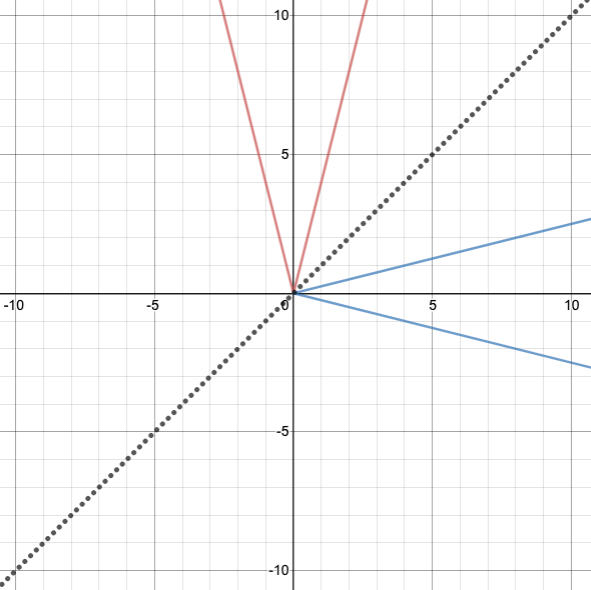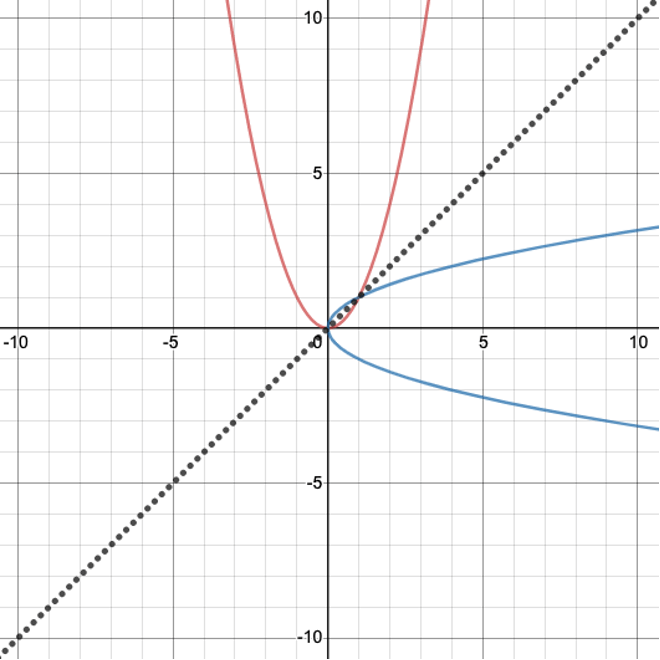# FNGR 2 | Lesson 5 | Practice (Graphing Inverse Functions)# Graphing Inverse Functions

Use Desmos to graph the following equations on the same axes.  Determine whether the two equations are inverse functions and state your rationale.

1.  $$y=2-3x$$ ; $$y=-\dfrac{1}{3}x +\dfrac23$$

2.  $$y=x^2-1$$ ; $$y=\mp \sqrt{x+1}$$

3.  $$y=|x|$$  and  $$x=|y|$$

4.  $$y=x^3+1$$ ; $$y=\sqrt{x-1}$$

5. $$y=\dfrac{3}{5} x-1$$ ; $$y=\dfrac{5}{3}x + \dfrac{5}{3}$$

Determine if the graphs shown in the figures below are inverses.  Explain your reasoning.

6.7.8.